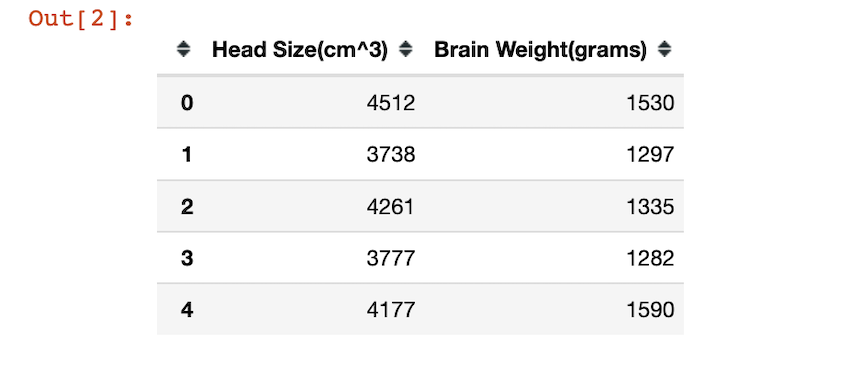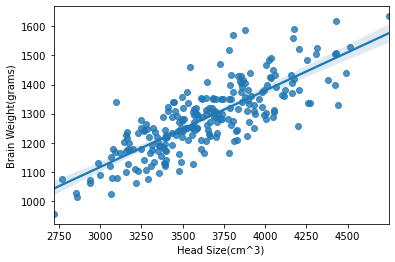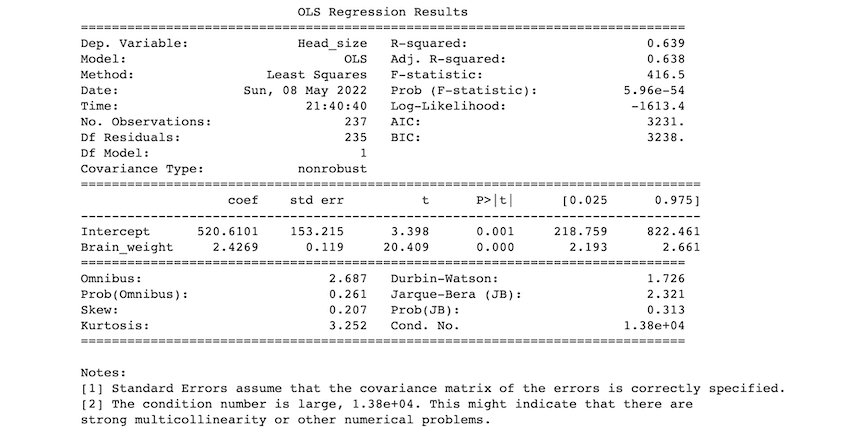GFG App
Open AppBrowser
Continue

# Linear Regression in Python using Statsmodels

In this article, we will discuss how to use statsmodels using Linear Regression in Python.

Linear regression analysis is a statistical technique for predicting the value of one variable(dependent variable) based on the value of another(independent variable). The dependent variable is the variable that we want to predict or forecast. In simple linear regression, there’s one independent variable used to predict a single dependent variable. In the case of multilinear regression, there’s more than one independent variable. The independent variable is the one you’re using to forecast the value of the other variable. The statsmodels.regression.linear_model.OLS method is used to perform linear regression. Linear equations are of the form:

``

Syntax: statsmodels.regression.linear_model.OLS(endog, exog=None, missing=’none’, hasconst=None, **kwargs)

Parameters:

• endog: array like object.
• exog: array like object.
• missing: str. None, decrease, and raise are the available alternatives. If the value is ‘none,’ no nan testing is performed. Any observations with nans are dropped if ‘drop’ is selected. An error is raised if ‘raise’ is used. ‘none’ is the default.
• hasconst: None or Bool. Indicates whether a user-supplied constant is included in the RHS. If True, k constant is set to 1 and all outcome statistics are calculated as if a constant is present. If False, k constant is set to 0 and no constant is verified.
• **kwargs: When using the formula interface, additional arguments are utilised to set model characteristics.

Return: Ordinary least squares are returned.

### Installation

```pip install numpy
pip install pandas
pip install statsmodels```

## Stepwise Implementation

Step 1: Import packages.

Importing the required packages is the first step of modeling. The pandas, NumPy, and stats model packages are imported.

```import numpy as np
import pandas as pd
import statsmodels.api as sm```

To access the CSV file click here. The CSV file is read using pandas.read_csv() method. The head or the first five rows of the dataset is returned by using the head() method. Head size and Brain weight are the columns.

## Python3

 `df ``=` `pd.read_csv(``'headbrain1.csv'``)` `df.head()`

The head of the data frame looks like this:Visualizing the data:

By using the matplotlib and seaborn packages, we visualize the data. sns.regplot() function helps us create a regression plot.

## Python3

 `# import packages` `import` `pandas as pd` `import` `matplotlib.pyplot as plt` `import` `seaborn as sns`   `df ``=` `pd.read_csv(``'headbrain1.csv'``)` `sns.regplot(``'Head Size(cm^3)'``, ``'Brain Weight(grams)'``, data``=``df)`   `plt.show()`

Output:Step 3: Setting a hypothesis.

• Null hypothesis (H0): There is no relationship between head size and brain weight.
• Alternative hypothesis (Ha): There is a relationship between head size and brain weight.

Step 4: Fitting the model

statsmodels.regression.linear_model.OLS() method is used to get ordinary least squares, and fit() method is used to fit the data in it. The ols method takes in the data and performs linear regression. we provide the dependent and independent columns in this format :

inpendent_columns ~ dependent_column:

left side of the ~ operator contains the independent variables and right side of the operator contains the name of the dependent variable or the predicted column.

## Python3

 `df.columns ``=` `[``'Head_size'``, ``'Brain_weight'``]` `model ``=` `smf.ols(formula``=``'Head_size ~ Brain_weight'``, data``=``df).fit()`

Step 5: Summary of the model.

All the summary statistics of the linear regression model are returned by the model.summary() method. The p-value and many other values/statistics are known by this method. Predictions about the data are found by the model.summary() method.

## Python3

 `print``(model.summary())`

## Python3

 `# import packages` `import` `numpy as np` `import` `pandas as pd` `import` `statsmodels.formula.api as smf`   `# loading the csv file` `df ``=` `pd.read_csv(``'headbrain1.csv'``)` `print``(df.head())`   `# fitting the model` `df.columns ``=` `[``'Head_size'``, ``'Brain_weight'``]` `model ``=` `smf.ols(formula``=``'Head_size ~ Brain_weight'``, data``=``df).fit()`   `# model summary` `print``(model.summary())`

Output:### Description of some of the terms in the table :

•  R- squared value: R-squared value ranges between 0 and 1. An R-squared of 100 percent indicates that all changes in the dependent variable are completely explained by changes in the independent variable(s). if we get 1 as an r-squared value it means there’s a perfect fit. In our example, the r-squared value is 0.638.
• F- statistic: The F statistic simply compares the combined effect of all variables. In simplest terms, reject the null hypothesis if your alpha level is greater than your p-value.
• coef: the coefficients of the independent variables in the regression equation.

### Our predictions:

If we take our significance level (alpha) to be 0.05, we reject the null hypothesis and accept the alternative hypothesis as p<0.05. so, we can say that there is a relationship between head size and brain weight.

My Personal Notes arrow_drop_up